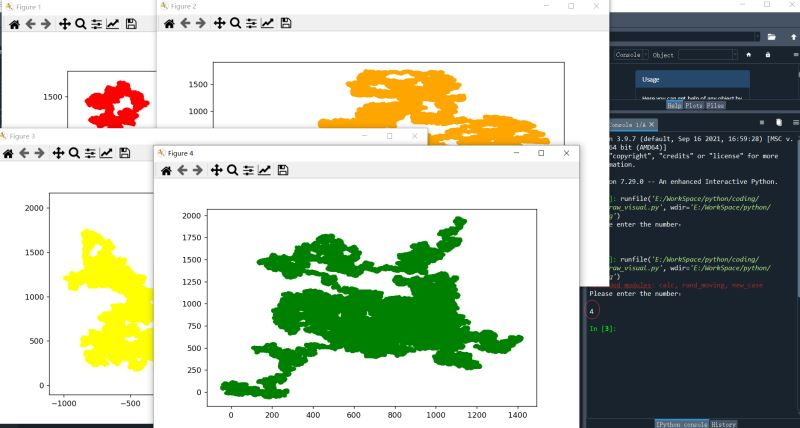# Python利用随机函数生成变化图形的方法

1、上述程序是否能进行优化（比如功能相同的）

2、创建三个3个实例，用了3个语句，能否建一个函数，只输入一个数n，就自动创建n个实例？同时，每个实例的num_times随机，(n比较大时，num_times应该比较小)

3、当实现上述功能后，程序运行，只输入一个参数（创建实例的个数），就会自动生成对应的num_times，并分别调用相关函数生成对应图表。

```x_direction = choice([1,-1])       #x的移动方向，1向上，0不变，-1向下
x_distance = choice([0,1,2,3,4,5]) #x的每次移动的像素，
x_step = x_direction*x_distance    #移动方向乘以移动距离，以确定沿x移动的距离

y_direction =  choice([1,-1])      #y的移动方向，1向上，0不变，-1向下
y_distance = choice([0,1,2,3,4,5]) #y的每次移动的像素，
y_step = y_direction*y_distance    #移动方向乘以移动距离，以确定沿y移动的距离```

```def get_step(self,direction,distance):
return distance*direction

def fill_moving(self):
while len(self.x_values)<self.num_times:#循环不断运行，直到漫步包含所需数量的点num_times
x_step = self.get_step(choice([1,-1]),choice([0,1,2,3,4,5]))     #直接调用get_step方法，使代码更加简洁
y_step = self.get_step(choice([1,-1]),choice([0,1,2,3,4,5]))```

1）要能生成实例个数n，且还有对应的移动次数num_times，可考虑用字典，即实例个数为key，对应移动次数num_times为键值,当输入2时，如  caselist={&rsquo;1&lsquo;:'150000'，&rsquo;2&lsquo;:'250000'}

2）读取字典每个项目，将对应的key和value传递给由Rand_moving类创建的实例，key的个数即为需要创建实例的个数，对应的value值为移动次数。

3）输入数字，即为字典key的个数，存入字典，同时利用随机函数生成一个num_times保存到对应的value中。

1，重新定义一个类 New_case() 作用：接收一个数据，并根据这个数据自动生成一个字典，

```class New_case():
#定义New_case类
def __init__(self,numbers): #定义要创建的实例个数
self.numbers=numbers
self.caselist={}  #定义一个空的caselist字典
self.case = 0
while self.case < self.numbers: #当变量case小于给定值时，
self.case += 1
times = choice([100000,150000,200000,250000])#随机选择一个移动次数
self.caselist[self.case] = times  #将value与key对应```

2、需要循环读取字典的key和value,并将value传递给类Rand_moving，随后再运行fill_moving()生成数据并保存到列表，随即用plt.scatter()进行绘图

```for key,value in self.caselist.items(): #字典不为空
colorkey=str(key)        #  将字典关键字转为字符串存到变量colorkey中
examplecase = Rand_moving(int(value))  #创建实例，将对应的value值传递类Rand_moving
examplecase.fill_moving()  #调用类Rand_moving中的方法fill_moving()计算移动相关数据并保存到列表中
plt.figure(dpi=128,figsize=(12, 10))   #创建画面屏幕
plt.scatter(examplecase.x_values,examplecase.y_values,c=self.colors[colorkey],s=15)
plt.show()```

```import matplotlib.pyplot as plt

from rand_moving import *

class New_case():
#定义New_case类
def __init__(self,numbers): #定义要创建的实例个数
self.numbers=numbers
self.caselist={}  #定义一个空的cases列表
self.case = 0    #定义一个case变量
self.colors={'1':'red','2':'orange','3':'yellow','4':'green','5':'blue','6':'puple'}#创建了一新字典colors{}，将生成的个数与颜色相对应

while self.case < self.numbers:  #小于给定实例个数时
self.case += 1
times = choice([100000,150000,200000,250000]) #随机生成一个移动次数
self.caselist[self.case] = times    #将变量case作为key, times作为value保存到字典中

def case_moving(self):  #重新定义一个方法，即访问字典所有项
for key,value in self.caselist.items(): #字典不为空
colorkey=str(key)        #  将字典关键字转为字符串存到变量colorkey中
examplecase = Rand_moving(int(value))  #创建实例，将对应的value值传递类Rand_moving
examplecase.fill_moving()  #调用类Rand_moving中的方法fill_moving()计算移动相关数据并保存到列表中
plt.figure(dpi=128,figsize=(12, 10))   #创建画面屏幕
plt.scatter(examplecase.x_values,examplecase.y_values,c=self.colors[colorkey],s=15)#注意调用了上述新字典的颜色
plt.show()```

3、主程序

```import matplotlib.pyplot as plt

from rand_moving import *
from new_case import *

n = input()   #将输入的数据保存到变量n中，注意所有输入均为字符串，

testcase = New_case(int(n)) #将n转为整型数据，创建实例个数
testcase.case_moving()```self.colors={'1':'red','2':'orange','3':'yellow','4':'green','5':'blue','6':'puple'} 注意：图的颜色分别与colors字典中对应。

```plt.axes().get_xaxis().set_visible(False)
plt.axes().get_yaxis().set_visible(False)```